# Relativity

## Homework Help & Tutoring

We offer an array of different online Relativity tutors, all of whom are advanced in their fields and highly qualified to instruct you.Send your subject help request Submit your homework problem, or a general tutoring request.
Get quotes from qualified tutors Receive a response from one of our tutors as soon as possible, sometimes within minutes!
Relativity Tutors Available Now
25 tutors available
Relativity Homework Library
17 total solutions
See what our students are saying
Can you help me with my homework in less than 24 hours?
Can you help me with my exam/quiz/test?
How much will it cost?
What kind of payments do you accept?

# Relativity

The Relativistic Doppler shift

Consider a source of very short-duration electromagnetic pulses (e.g., a radar antenna) at rest at the origin of reference frame S. An observer moves in the x-direction at velocity v as seen in frame S. The observer is at rest in frame S’. Suppose a first pulse is sent at t = 0 when the observer is at position x = xo Further suppose the (n + 1)th pulse is sent out at t = nτ where  τ is the period.

1. Carefully draw the Minkowski diagram (space-time diagram) for frame S identifying the two events representing the arrival of the first and (n + 1)th pulse at the observer.
2. Find the time interval between those two pulses in the observer’s frame S’.
3. And therefore find the frequency ν' in the observer’s frame in terms of the source frequency ν and the observer’s velocity factor β. This is the relativistic Doppler shift.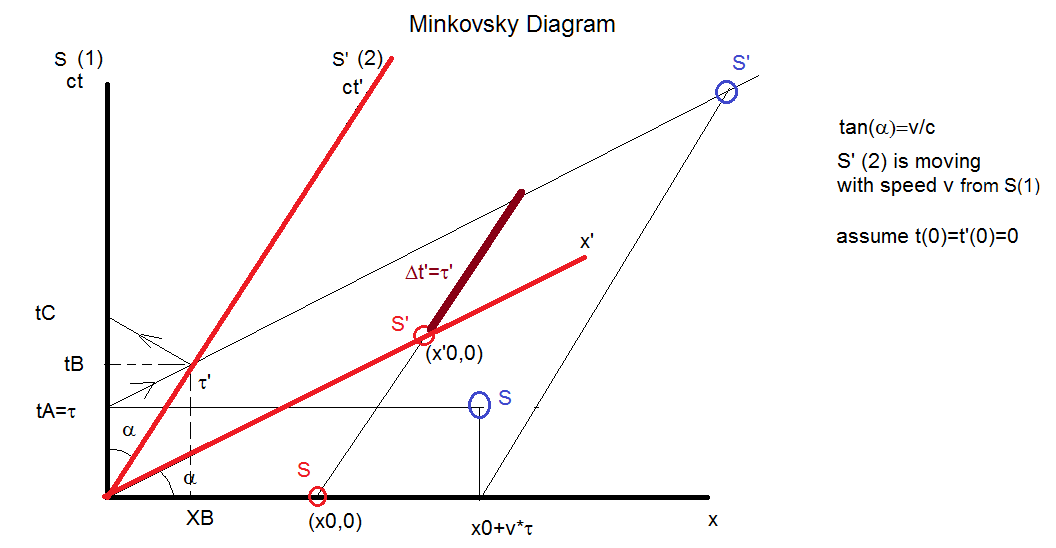S’(2) is moving right with a speed v. S(1) is standing. At (X0,0) in S the first light is emitted. These events are the red circles in the S and S’ axes. At (X0 + ντ,τ) in S' the second light is emitted. These events are the blue circles in S and S’.  The interval is Δt= τ' in S' (the brown segment).  In S the interval is Δt = τ. Thus τ is the time between two consecutive emissions of light.

From the diagram we can immediately deduce that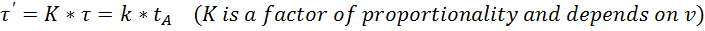The speed v of S’ moving is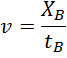To find tB, suppose one has a mirror at τ' that reflects back the light emitted at tA = τ towards S. This light arrives in S at tC  = K * τ' (from symmetry considerations). Therefore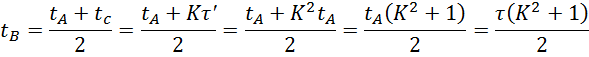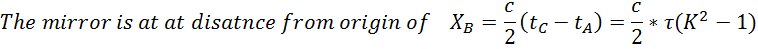Therefore the speed of S’ is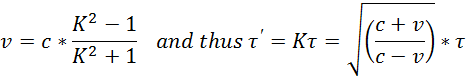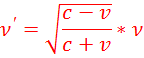## College Relativity Homework Help

Since we have tutors in all Relativity related topics, we can provide a range of different services. Our online Relativity tutors will:

• Provide specific insight for homework assignments.
• Review broad conceptual ideas and chapters.
• Simplify complex topics into digestible pieces of information.
• Answer any Relativity related questions.
• Tailor instruction to fit your style of learning.

With these capabilities, our college Relativity tutors will give you the tools you need to gain a comprehensive knowledge of Relativity you can use in future courses.